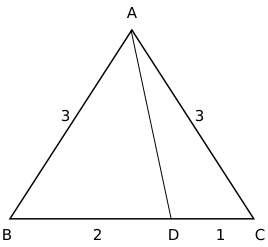Question 22

# The length of each side of an equilateral triangle ABC is 3 cm. Let D be a point on BC such that the area of triangle ADC is half the area of triangle ABD. Then the length of AD, in cm, is

SolutionArea of triangle ABD is twice the area of triangle ACD

$$\angle\ ADB=\theta\$$

$$\frac{1}{2}\left(AD\right)\left(BD\right)\sin\theta\ =2\left(\frac{1}{2}\left(AD\left(CD\right)\sin\left(180-\theta\ \right)\right)\right)$$

$$BD\ =2CD$$

Therefore, BD = 2 and CD = 1

$$\angle\ ABC=\angle\ ACB=60^{\circ\ }$$

Applying cosine rule in triangle ADC, we get

$$\cos\angle\ ACD=\ \frac{\ AC^2+CD^2-AD^2}{2\left(AC\right)\left(CD\right)}$$

$$\frac{1}{2}=\ \frac{\ 9+1-AD^2}{6}$$

$$AD^2=7$$

$$AD=\sqrt{\ 7}$$